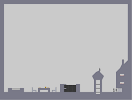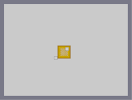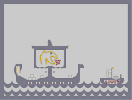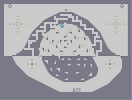### MamoswineHover over the thumbnail for a full-size version.

Author BluePretzel author:bluepretzel colour mamoswine n-art nart pokemon rated 2009-12-22 2009-12-22 4 by 103 people. \$Mamoswine#BluePretzel#n-art#1I0000000000000000000F1A000000000000000000000>000000000000000000000000000000000000000000000000000000000000000000000000000000000000000000000000000000000000000000000000000000000000000000000000000000000000000000000000000000000000000000000000000000000000000000000000000000000000000000000000000000000000000000000000000000000000000000000000000000000000000000000000000000000000000000000000000000000000000000000000000000000000000000000000000000000000000000000000000000000000000000000000000000000000000000000000000000000000000000000000000000000000000000000000000000000000000000000000000000000000000000000000000000000000000000000000000000000000000000000000000000000000000000000@000000000000000000000?1H0000000000000000000G1|12^666,570!12^666,558!12^660,546!12^660,534!12^660,522!12^654,510!12^654,498!12^660,492!12^666,480!12^672,474!12^660,474!12^672,462!12^660,456!12^648,444!12^648,432!12^642,420!12^642,408!12^636,396!12^630,384!12^630,372!12^624,360!12^618,348!12^612,336!12^606,324!12^600,312!12^594,300!12^582,288!12^576,276!12^564,264!12^552,252!12^540,240!12^528,228!12^516,222!12^504,216!12^492,210!12^480,204!12^468,198!12^456,198!12^444,192!12^432,192!12^420,192!12^408,192!12^396,192!12^384,198!12^372,198!12^360,204!12^348,210!12^336,216!12^324,216!12^312,216!12^300,228!12^288,240!12^282,252!12^270,258!12^258,270!12^246,282!12^240,294!12^240,306!12^246,318!12^246,330!12^252,342!12^264,336!12^264,324!12^270,318!12^282,318!12^270,306!12^270,294!12^270,282!12^276,270!12^282,264!12^288,258!12^294,252!12^300,246!12^306,240!12^318,240!12^324,246!12^330,252!12^336,258!12^348,264!12^360,264!12^372,264!12^384,252!12^396,246!12^408,240!12^420,234!12^432,234!12^444,240!12^456,252!12^468,258!12^474,270!12^480,312!12^468,318!12^456,324!12^444,330!12^426,330!12^414,324!12^408,312!12^396,306!12^384,294!12^372,288!12^360,282!12^348,282!12^336,282!12^324,294!12^300,312!12^276,336!12^276,348!12^276,360!12^276,372!12^282,384!12^294,378!12^306,378!12^318,378!12^330,384!12^342,378!12^348,366!12^342,354!12^342,342!12^330,330!12^324,324!12^312,318!12^462,336!12^456,348!12^468,348!12^480,354!12^474,366!12^462,366!12^474,378!12^480,384!12^468,384!12^474,396!12^480,402!12^468,402!12^468,414!12^474,420!12^474,432!12^462,432!12^456,426!12^462,438!12^456,444!12^450,438!12^438,432!12^438,444!12^438,456!12^426,456!12^426,444!12^420,456!12^414,456!12^408,450!12^408,462!12^402,468!12^354,450!12^348,468!12^336,456!12^330,444!12^330,462!12^318,456!12^312,450!12^306,438!12^300,450!12^294,456!12^288,444!12^282,450!12^276,456!12^264,438!12^270,450!12^252,444!12^240,450!12^240,438!12^240,426!12^228,426!12^234,414!12^246,408!12^252,402!12^240,402!12^246,390!12^252,384!12^240,378!12^228,378!12^234,366!12^246,360!12^234,354!12^240,348!12^228,342!12^222,354!12^216,366!12^216,378!12^216,390!12^216,402!12^216,414!12^216,426!12^210,438!12^204,450!12^198,468!12^198,480!12^204,492!12^198,510!12^186,522!12^174,528!12^156,534!12^138,528!12^126,522!12^114,516!12^96,492!12^90,474!12^84,456!12^78,438!12^78,420!12^84,408!12^84,390!12^72,396!12^60,408!12^54,426!12^48,444!12^42,462!12^36,480!12^36,498!12^36,516!12^42,534!12^60,564!12^72,576!12^234,576!12^246,564!12^252,552!12^264,540!12^258,528!12^252,516!12^246,504!12^240,492!12^234,480!12^222,474!12^216,486!12^288,564!12^300,570!12^312,576!12^372,576!12^366,558!12^366,540!12^360,522!12^360,504!12^360,486!12^360,468!12^360,450!12^366,432!12^372,414!12^378,396!12^384,384!12^390,402!12^390,420!12^396,438!12^396,456!12^402,486!12^408,498!12^414,510!12^420,522!12^426,534!12^432,546!12^444,552!12^432,522!12^432,510!12^432,498!12^432,486!12^432,474!12^438,462!12^450,450!12^468,450!12^480,456!12^492,462!12^504,474!12^510,486!12^510,498!12^510,510!12^510,522!12^510,534!12^510,546!12^510,558!12^504,570!12^516,510!12^522,498!12^528,486!12^534,456!12^528,438!12^534,468!12^576,462!12^576,474!12^576,486!12^576,498!12^582,510!12^582,522!12^588,540!12^588,552!12^588,564!12^588,576!12^606,576!12^612,570!12^618,558!12^624,546!12^630,534!12^636,522!12^439,253!12^446,253!12^451,256!12^455,262!12^459,272!12^461,282!12^451,300!12^440,306!12^427,302!12^419,297!12^409,290!12^403,280!12^399,274!12^313,264!12^322,271!12^322,281!12^315,289!12^298,299!12^286,298!12^280,288!12^283,275!12^288,265!12^436,304!12^348,222!12^360,222!12^372,222!12^384,216!12^396,210!12^414,204!12^432,204!12^444,210!12^456,216!12^468,222!12^480,228!12^492,234!12^504,246!12^516,258!12^528,270!12^540,282!12^546,294!12^546,306!12^540,318!12^534,330!12^522,342!12^510,354!12^504,366!12^492,372!12^480,372!12^534,480!12^532,448!12^535,474!12^535,461!12^519,504!12^507,562!12^502,575!12^510,553!12^510,540!12^510,531!12^510,517!12^510,503!12^509,492!12^508,480!12^498,467!12^486,459!12^475,454!12^462,448!12^239,432!12^239,443!12^246,447!12^257,441!12^267,445!12^273,450!12^280,452!12^291,445!12^291,451!12^291,455!12^303,444!12^310,444!12^323,458!12^330,455!12^330,450!12^342,456!12^343,463!12^352,465!12^353,459!12^353,455!12^380,390!12^388,393!12^374,405!12^368,422!12^362,441!12^361,458!12^360,476!12^361,494!12^359,513!12^363,532!12^367,549!12^368,568!12^438,550!12^429,539!12^424,528!12^418,516!12^412,505!12^406,492!12^400,476!12^398,463!12^396,447!12^393,430!12^390,410!12^80,392!12^66,401!12^57,416!12^52,435!12^45,452!12^38,470!12^36,489!12^36,508!12^39,525!12^46,542!12^54,559!12^67,570!12^85,399!12^80,414!12^76,429!12^80,447!12^87,465!12^92,481!12^100,498!12^111,510!12^119,521!12^105,505!12^94,488!12^133,527!12^147,532!12^167,531!12^179,525!12^191,517!12^203,503!12^210,495!12^219,479!12^227,477!12^237,487!12^243,499!12^249,511!12^256,523!12^261,534!12^259,545!12^249,557!12^241,570!12^280,558!12^293,569!12^305,573!12^274,554!12^267,549!12^199,488!12^198,475!12^199,459!12^205,445!12^214,432!12^233,427!12^230,420!12^239,411!12^244,406!12^245,400!12^250,396!12^248,385!12^246,380!12^233,380!12^230,371!12^240,364!12^239,358!12^236,352!12^245,344!12^258,338!12^458,340!12^461,350!12^473,351!12^478,360!12^466,366!12^477,374!12^471,382!12^474,385!12^480,389!12^476,391!12^477,397!12^475,403!12^470,408!12^477,424!12^469,432!12^460,429!12^430,531!12^431,516!12^433,506!12^431,493!12^431,481!12^433,467!12^442,451!12^452,447!12^444,434!12^438,438!12^431,446!12^427,450!12^410,443!12^407,457!12^404,464!12^287,380!12^278,378!12^277,367!12^274,355!12^276,341!12^275,327!12^278,322!12^287,315!12^292,312!12^304,315!12^317,321!12^337,336!12^341,346!12^344,360!12^346,373!12^337,380!12^323,381!12^312,377!12^300,377!12^325,275!12^216,421!12^216,408!12^216,399!12^216,383!12^215,372!12^217,360!12^224,346!12^230,336!12^246,324!12^244,312!12^238,300!12^241,286!12^254,275!12^249,276!12^264,264!12^275,255!12^285,247!12^293,232!12^305,221!12^318,217!12^330,215!12^342,214!12^354,207!12^365,200!12^378,197!12^389,194!12^401,192!12^413,190!12^425,193!12^439,192!12^451,195!12^460,197!12^473,201!12^487,207!12^498,213!12^509,219!12^521,225!12^534,233!12^546,245!12^558,259!12^571,271!12^580,282!12^587,294!12^598,305!12^604,319!12^608,329!12^615,342!12^620,353!12^627,365!12^631,378!12^634,391!12^641,403!12^643,414!12^646,426!12^649,439!12^654,450!12^668,460!12^656,454!12^671,468!12^669,477!12^663,478!12^660,484!12^657,493!12^653,504!12^657,516!12^660,527!12^658,540!12^664,552!12^667,563!12^634,527!12^627,540!12^620,552!12^614,565!12^610,573!12^586,569!12^587,560!12^587,549!12^586,534!12^584,529!12^583,519!12^579,505!12^576,493!12^576,482!12^575,469!12^497,371!12^486,373!12^507,361!12^516,347!12^528,335!12^537,323!12^542,313!12^546,300!12^544,289!12^533,276!12^522,263!12^510,251!12^498,240!12^487,232!12^474,226!12^462,218!12^451,212!12^438,206!12^421,204!12^404,206!12^426,204!12^391,213!12^378,219!12^366,222!12^355,222!12^342,219!12^264,327!12^268,320!12^270,312!12^270,299!12^267,286!12^271,276!12^312,239!12^342,261!12^354,264!12^366,264!12^380,258!12^390,246!12^403,244!12^413,236!12^426,234!12^437,238!12^451,244!12^461,254!12^471,263!12^479,277!12^486,306!12^474,315!12^462,320!12^450,326!12^434,332!12^421,328!12^409,318!12^402,309!12^391,300!12^378,291!12^367,286!12^356,284!12^342,282!12^332,288!12^318,298!12^289,300!12^279,293!12^278,281!12^401,278!12^404,279!12^404,281!12^406,283!12^406,284!12^408,286!12^412,290!12^414,292!12^416,294!12^416,295!12^418,297!12^422,299!12^425,300!12^427,301!12^429,301!12^433,304!12^435,305!12^439,305!12^442,305!12^448,304!12^448,302!12^450,301!12^453,300!12^456,297!12^458,294!12^461,289!12^462,285!12^462,282!12^462,280!12^462,276!12^461,275!12^457,270!12^456,266!12^456,265!12^446,255!12^444,254!12^443,253!12^304,255!12^304,255!12^297,259!12^296,259!12^295,261!12^288,268!12^281,277!12^281,279!12^281,281!12^281,287!12^310,293!12^311,290!12^314,289!12^315,286!12^316,287!12^319,285!12^319,282!12^322,281!12^324,279!12^324,275!12^325,274!12^324,271!12^322,269!12^320,268!12^317,266!12^316,266!12^314,264!12^312,262!12^311,261!12^309,259!12^308,258!12^306,255!12^305,255!12^440,252!12^435,252!12^431,252!12^428,254!12^425,256!12^420,256!12^419,258!12^421,257!12^416,258!12^414,260!12^412,261!12^409,261!12^407,263!12^404,264!12^402,266!12^398,268!12^396,269!12^397,272!12^399,274!12^264,332!12^276,333!12^241,318!12^240,323!12^237,328!12^233,332!12^314,301!12^305,307!12^310,304!12^269,289!12^304,242!12^246,336!12^246,342!0^204,468!0^210,462!0^210,474!0^216,468!0^204,480!0^210,486!0^216,456!0^222,462!0^204,456!0^210,450!0^222,450!0^228,456!0^216,444!0^228,444!0^222,438!0^222,426!0^222,414!0^222,402!0^222,390!0^222,378!0^222,366!0^228,360!0^228,348!0^234,342!0^240,336!0^228,384!0^228,396!0^228,408!0^234,390!0^234,402!0^240,396!0^240,384!0^228,432!0^234,450!0^234,438!0^228,468!0^234,462!0^240,456!0^234,474!0^240,468!0^246,462!0^252,456!0^258,450!0^258,462!0^252,468!0^246,474!0^240,480!0^246,486!0^252,480!0^258,474!0^264,468!0^270,462!0^264,456!0^276,468!0^270,474!0^264,480!0^258,486!0^252,492!0^258,498!0^270,498!0^282,498!0^294,498!0^306,498!0^318,498!0^330,498!0^342,498!0^354,498!0^348,492!0^336,492!0^324,492!0^312,492!0^300,492!0^276,492!0^264,492!0^270,486!0^282,486!0^294,486!0^306,486!0^318,486!0^330,486!0^342,486!0^354,486!0^348,480!0^336,480!0^324,480!0^312,480!0^300,480!0^288,480!0^276,480!0^282,474!0^294,474!0^306,474!0^318,474!0^330,474!0^342,474!0^354,474!0^288,468!0^282,462!0^300,468!0^312,468!0^324,468!0^336,468!0^306,462!0^294,462!0^318,462!0^300,456!0^306,450!0^312,456!0^252,504!0^264,504!0^276,504!0^288,504!0^300,504!0^312,504!0^324,504!0^336,504!0^348,504!0^420,504!0^414,498!0^426,498!0^420,492!0^414,486!0^426,486!0^408,480!0^420,480!0^414,474!0^426,474!0^420,468!0^426,462!0^414,462!0^444,444!0^432,456!0^492,456!0^486,450!0^498,450!0^480,444!0^492,444!0^504,444!0^468,444!0^474,438!0^486,438!0^498,438!0^510,438!0^522,438!0^516,432!0^504,432!0^492,432!0^480,432!0^486,426!0^498,426!0^510,426!0^522,426!0^516,420!0^504,420!0^492,420!0^480,420!0^486,414!0^498,414!0^510,414!0^522,414!0^528,420!0^528,432!0^534,426!0^534,414!0^528,408!0^516,408!0^504,408!0^492,408!0^480,408!0^474,414!0^540,408!0^540,396!0^540,384!0^540,420!0^540,432!0^552,432!0^564,432!0^570,414!0^564,408!0^570,402!0^570,390!0^552,384!0^552,408!0^522,402!0^510,402!0^498,402!0^486,402!0^492,396!0^504,396!0^516,396!0^528,396!0^534,438!0^546,438!0^558,438!0^570,438!0^570,426!0^582,390!0^582,402!0^594,390!0^606,390!0^612,396!0^612,408!0^606,414!0^594,414!0^582,414!0^582,426!0^582,438!0^576,432!0^576,420!0^576,408!0^576,396!0^564,384!0^576,384!0^570,378!0^558,378!0^546,378!0^534,378!0^534,390!0^522,390!0^528,384!0^516,384!0^510,390!0^498,390!0^486,390!0^492,384!0^504,384!0^510,378!0^498,378!0^522,378!0^516,372!0^528,372!0^540,372!0^552,372!0^564,372!0^576,372!0^588,372!0^600,372!0^612,372!0^624,372!0^618,378!0^606,378!0^594,378!0^582,378!0^588,384!0^600,384!0^612,384!0^546,390!0^558,390!0^564,396!0^558,402!0^546,402!0^552,396!0^534,402!0^546,414!0^558,414!0^564,420!0^558,426!0^546,426!0^552,420!0^588,396!0^600,396!0^606,402!0^600,408!0^588,408!0^594,402!0^618,390!0^624,384!0^624,396!0^618,402!0^630,402!0^624,408!0^630,414!0^624,420!0^630,426!0^624,432!0^630,438!0^588,420!0^600,420!0^612,420!0^618,414!0^618,426!0^606,426!0^594,426!0^588,432!0^600,432!0^612,432!0^618,438!0^606,438!0^594,438!0^540,444!0^552,444!0^564,444!0^576,444!0^588,444!0^600,444!0^612,444!0^624,444!0^636,444!0^636,432!0^636,420!0^636,408!0^618,366!0^606,366!0^594,366!0^582,366!0^570,366!0^558,366!0^546,366!0^534,366!0^522,366!0^510,366!0^504,372!0^486,378!0^642,438!0^516,360!0^528,360!0^540,360!0^552,360!0^564,360!0^576,360!0^588,360!0^600,360!0^612,360!0^606,354!0^612,348!0^594,354!0^582,354!0^570,354!0^558,354!0^546,354!0^534,354!0^522,354!0^528,348!0^540,348!0^552,348!0^564,348!0^576,348!0^588,348!0^600,348!0^606,342!0^594,342!0^582,342!0^570,342!0^558,342!0^546,342!0^534,342!0^540,336!0^552,336!0^564,336!0^576,336!0^588,336!0^600,336!0^594,330!0^582,330!0^570,330!0^558,330!0^546,330!0^552,324!0^564,324!0^576,324!0^588,324!0^600,324!0^594,318!0^582,318!0^570,318!0^558,318!0^546,318!0^552,312!0^564,312!0^576,312!0^588,312!0^582,306!0^570,306!0^558,306!0^564,300!0^576,300!0^588,300!0^552,300!0^558,294!0^570,294!0^582,294!0^564,288!0^576,288!0^552,288!0^558,282!0^570,282!0^546,282!0^564,276!0^552,276!0^540,276!0^546,270!0^558,270!0^552,264!0^540,264!0^534,270!0^534,258!0^546,258!0^528,264!0^528,252!0^540,252!0^522,258!0^516,252!0^522,246!0^534,246!0^528,240!0^516,240!0^504,240!0^510,246!0^510,234!0^522,234!0^516,228!0^504,228!0^498,234!0^498,222!0^486,222!0^492,228!0^492,216!0^480,216!0^468,216!0^474,210!0^486,210!0^474,222!0^462,210!0^468,204!0^456,204!0^444,204!0^450,198!0^438,198!0^426,198!0^414,198!0^402,198!0^390,198!0^396,204!0^384,204!0^372,204!0^378,210!0^372,216!0^360,216!0^366,210!0^354,210!0^348,216!0^390,210!0^642,426!0^582,450!0^582,462!0^582,474!0^582,486!0^582,498!0^588,492!0^588,480!0^588,468!0^588,456!0^594,450!0^606,450!0^618,450!0^630,450!0^642,450!0^594,462!0^600,456!0^612,456!0^624,456!0^636,456!0^648,456!0^630,462!0^618,462!0^606,462!0^600,468!0^594,474!0^594,486!0^600,480!0^612,468!0^624,468!0^606,474!0^546,450!0^558,450!0^570,450!0^576,456!0^564,456!0^552,456!0^540,456!0^546,462!0^558,462!0^570,462!0^564,468!0^570,474!0^570,486!0^570,498!0^564,480!0^558,474!0^552,468!0^564,492!0^558,486!0^552,480!0^546,474!0^540,468!0^270,510!0^282,510!0^294,510!0^306,510!0^318,510!0^330,510!0^342,510!0^312,516!0^300,516!0^288,516!0^324,516!0^276,516!0^336,516!3^288,390!3^300,390!3^312,390!3^324,390!3^336,390!3^336,402!3^336,414!3^324,414!3^324,402!3^312,402!3^300,402!3^312,414!3^348,402!3^348,390!0^330,396!0^342,396!0^318,396!0^306,396!0^294,396!0^294,408!0^306,408!0^318,408!0^330,408!0^342,408!0^354,408!0^354,396!0^354,384!0^342,384!0^318,384!0^306,384!0^294,384!0^282,396!0^282,408!0^294,420!0^306,420!0^318,420!0^330,420!0^342,420!0^354,420!0^366,408!0^366,396!0^366,384!0^354,372!0^366,372!0^366,360!0^354,360!0^354,348!0^366,348!0^366,336!0^354,336!0^354,324!0^342,324!0^342,312!0^330,312!0^318,312!0^330,324!0^330,300!0^342,300!0^342,288!0^354,288!0^354,300!0^354,312!0^366,300!0^378,300!0^378,312!0^366,312!0^366,324!0^378,324!0^378,336!0^378,348!0^378,360!0^378,372!0^378,384!0^390,372!0^390,360!0^390,348!0^390,336!0^390,324!0^390,312!0^402,324!0^402,336!0^402,348!0^402,360!0^402,372!0^414,348!0^414,336!0^426,336!0^426,348!0^438,348!0^438,336!0^450,336!0^450,348!0^450,360!0^438,360!0^426,360!0^414,360!0^414,372!0^426,372!0^438,372!0^450,372!0^462,360!0^474,360!0^462,372!0^474,372!0^462,384!0^462,396!0^450,396!0^450,384!0^438,384!0^426,384!0^414,384!0^402,384!0^390,384!0^402,396!0^414,396!0^426,396!0^438,396!0^402,408!0^414,408!0^426,408!0^438,408!0^450,408!0^450,420!0^438,420!0^426,420!0^414,420!0^402,420!0^402,432!0^414,432!0^426,432!0^450,432!0^462,420!0^462,408!0^414,444!0^402,444!0^402,456!0^354,432!0^342,432!0^330,432!0^318,432!0^306,432!0^294,432!0^282,432!0^282,420!0^270,420!0^270,408!0^270,396!0^270,384!0^270,372!0^270,360!0^270,348!0^270,336!0^270,324!0^258,348!0^246,348!0^258,360!0^258,372!0^246,372!0^258,384!0^258,396!0^258,408!0^258,420!0^246,420!0^246,432!0^258,432!0^270,432!0^276,444!0^318,444!0^342,444!0^354,444!3^210,468!3^216,462!3^210,456!3^222,456!3^234,456!3^246,456!3^258,456!3^270,456!3^282,456!3^306,456!3^336,462!3^324,462!3^312,462!3^300,462!3^288,462!3^276,462!3^264,462!3^252,462!3^240,462!3^228,462!3^234,468!3^222,468!3^222,444!3^234,444!3^210,444!3^216,450!3^228,450!3^228,438!3^216,438!3^222,432!3^222,420!3^222,408!3^222,396!3^222,384!3^222,372!3^222,360!3^228,354!3^234,348!3^234,336!3^240,330!3^240,342!3^234,360!3^228,366!3^234,384!3^234,396!3^234,408!3^228,414!3^228,402!3^228,390!3^240,390!3^210,480!3^204,474!3^204,462!3^204,486!3^210,492!3^228,474!3^240,474!3^246,468!3^258,468!3^270,468!3^282,468!3^294,468!3^306,468!3^318,468!3^330,468!3^342,468!3^354,468!3^348,474!3^336,474!3^324,474!3^312,474!3^300,474!3^288,474!3^276,474!3^264,474!3^252,474!3^246,480!3^258,480!3^270,480!3^282,480!3^294,480!3^306,480!3^318,480!3^330,480!3^342,480!3^354,480!3^348,486!3^336,486!3^324,486!3^312,486!3^300,486!0^288,492!3^288,486!3^276,486!3^264,486!3^252,486!3^240,486!3^246,492!3^252,498!3^258,492!3^270,492!3^282,492!3^294,492!3^306,492!3^264,498!3^276,498!3^288,498!3^300,498!3^312,498!3^318,492!3^330,492!3^342,492!3^354,492!3^348,498!3^336,498!3^324,498!3^258,504!3^270,504!3^282,504!3^294,504!3^306,504!3^318,504!3^330,504!3^342,504!3^354,504!3^336,510!3^324,510!3^312,510!3^300,510!3^288,510!3^276,510!3^282,516!3^294,516!3^306,516!3^318,516!3^330,516!3^234,432!3^228,426!0^408,468!3^420,474!3^420,462!3^408,474!3^420,486!3^426,468!3^414,468!3^414,480!3^426,480!3^414,492!3^408,486!3^426,492!3^420,498!3^426,504!3^438,450!3^432,462!3^444,438!3^450,444!3^474,444!3^486,444!3^498,444!3^492,438!3^480,438!3^468,438!3^486,432!3^498,432!3^510,432!3^522,432!3^534,432!3^546,432!3^558,432!3^570,432!3^582,432!3^594,432!3^606,432!3^618,432!3^630,432!3^642,432!3^534,372!3^534,384!3^534,396!3^534,408!3^534,360!3^546,360!3^558,360!3^564,366!3^564,378!3^558,384!3^564,390!3^564,402!3^558,408!3^546,408!3^546,384!3^582,360!3^582,372!3^582,384!3^582,396!3^582,408!3^594,360!3^606,360!3^612,366!3^612,378!3^606,384!3^594,384!3^504,438!3^492,450!3^480,450!3^486,456!3^516,438!3^540,438!3^552,438!3^564,438!3^576,438!3^588,438!3^600,438!3^612,438!3^624,438!3^636,438!3^642,444!3^630,444!3^618,444!3^606,444!3^594,444!3^582,444!3^570,444!3^558,444!3^546,444!3^534,444!3^540,450!3^552,450!3^564,450!3^576,450!3^588,450!3^600,450!3^612,450!3^624,450!3^636,450!3^648,450!3^642,456!3^630,456!3^618,456!3^606,456!3^594,456!3^582,456!3^570,456!3^558,456!3^546,456!3^540,462!3^552,462!3^564,462!3^570,468!3^558,468!3^546,468!3^552,474!3^564,474!3^570,480!3^558,480!3^564,486!3^570,492!3^588,462!3^600,462!3^612,462!3^624,462!3^606,468!3^594,468!3^582,468!3^588,474!3^600,474!3^582,480!3^594,480!3^588,486!3^582,492!3^618,468!3^564,426!3^552,426!3^540,426!3^528,426!3^516,426!3^504,426!3^492,426!3^480,426!3^576,426!3^588,426!3^600,426!3^612,426!3^624,426!3^636,426!3^636,414!3^624,414!3^612,414!3^600,414!3^630,420!3^618,420!3^606,420!3^594,420!3^582,420!3^570,420!3^558,420!3^546,420!3^534,420!3^522,420!3^510,420!3^498,420!3^486,420!3^480,414!3^492,414!3^504,414!3^516,414!3^528,414!3^540,414!3^552,414!3^564,414!3^576,414!3^588,414!3^594,408!3^606,408!3^618,408!3^630,408!3^570,408!3^522,408!3^510,408!3^498,408!3^486,408!3^474,408!3^486,396!3^498,396!3^510,396!3^510,384!3^510,372!3^498,384!3^486,384!3^492,378!3^504,378!3^492,390!3^504,390!3^492,402!3^504,402!3^516,402!3^516,390!3^516,378!3^528,378!3^528,390!3^528,402!3^522,396!3^522,384!3^540,402!3^552,402!3^576,402!3^588,402!3^600,402!3^612,402!3^624,402!3^636,402!3^630,396!3^618,396!3^606,396!3^594,396!3^570,396!3^558,396!3^546,396!3^540,390!3^552,390!3^576,390!3^588,390!3^600,390!3^612,390!3^624,390!3^624,378!3^618,384!3^600,378!3^588,378!3^576,378!3^552,378!3^540,378!3^570,384!3^618,372!3^606,372!3^594,372!3^570,372!3^558,372!3^546,372!3^522,372!3^516,366!3^528,366!3^540,366!3^552,366!3^576,366!3^588,366!3^600,366!3^624,366!3^618,360!3^570,360!3^522,360!3^510,360!3^516,354!3^528,354!3^540,354!3^552,354!3^564,354!3^576,354!3^588,354!3^600,354!3^612,354!3^606,348!3^594,348!3^582,348!3^570,348!3^558,348!3^546,348!3^534,348!3^522,348!3^540,342!3^552,342!3^564,342!3^576,342!3^588,342!3^600,342!3^612,342!3^606,336!3^594,336!3^582,336!3^570,336!3^558,336!3^546,336!3^534,336!3^540,330!3^552,330!3^564,330!3^576,330!3^588,330!3^600,330!3^594,324!3^582,324!3^570,324!3^558,324!3^546,324!3^552,318!3^564,318!3^576,318!3^588,318!3^600,318!3^594,312!3^582,312!3^570,312!3^558,312!3^546,312!3^552,306!3^564,306!3^576,306!3^588,306!3^582,300!3^570,300!3^558,300!3^546,288!3^552,294!3^564,294!3^576,294!3^570,288!3^558,288!3^552,282!3^564,282!3^576,282!3^570,276!3^558,276!3^546,276!3^552,270!3^540,270!3^564,270!3^558,264!3^546,264!3^534,264!3^540,258!3^528,258!3^552,258!3^546,252!3^534,252!3^522,252!3^516,246!3^528,246!3^540,246!3^534,240!3^522,240!3^510,240!3^504,234!3^516,234!3^528,234!3^510,228!3^498,228!3^486,228!3^504,222!3^492,222!3^480,222!3^498,216!3^486,216!3^474,216!3^462,216!3^480,210!3^468,210!3^456,210!3^450,204!3^462,204!3^474,204!3^444,198!3^432,198!3^420,198!3^408,198!3^396,198!3^390,204!3^378,204!3^366,204!3^402,204!3^384,210!3^372,210!3^360,210!3^354,216!3^366,216!3^378,216!3^342,216!3^426,204!3^408,204!3^438,204!3^66,426!3^66,438!3^66,450!3^66,462!3^54,462!3^54,474!3^66,474!3^54,486!3^66,486!3^66,498!3^54,498!3^54,510!3^66,510!3^54,522!3^66,522!3^78,522!3^66,534!3^78,534!3^78,546!3^90,546!3^90,534!3^90,558!3^102,558!3^102,546!3^72,414!3^102,570!3^114,558!3^114,546!3^114,570!3^126,570!3^126,558!3^138,558!3^138,570!3^150,570!3^162,570!3^162,558!3^150,558!3^174,558!3^174,570!3^186,570!3^186,558!3^198,558!3^210,558!3^210,546!3^222,546!3^234,534!3^198,570!3^210,570!3^222,570!3^222,558!3^234,558!3^234,546!3^246,546!3^246,534!3^240,522!3^378,420!3^378,432!3^378,444!3^378,456!3^384,408!3^378,468!3^390,468!3^390,480!3^390,492!3^390,504!3^402,504!3^378,480!3^390,516!3^402,516!3^402,528!3^414,528!3^402,540!3^414,540!3^414,552!3^402,552!3^426,552!3^414,564!3^426,564!3^438,564!3^438,576!3^426,576!3^414,576!3^450,576!3^450,564!3^462,576!3^474,576!3^486,576!3^486,564!3^498,564!3^498,552!3^486,552!3^498,540!3^498,528!3^498,516!3^498,504!3^498,492!3^498,480!3^486,540!3^486,528!3^474,564!3^486,516!3^486,504!3^486,492!3^486,480!3^486,468!3^474,552!3^462,564!12^474,540!12^480,546!12^486,552!3^78,510!3^390,456!3^390,444!3^390,432!3^486,366!3^498,366!3^498,354!3^486,354!3^486,342!3^498,342!3^510,342!3^510,330!3^522,330!3^522,318!3^534,318!3^534,306!3^534,294!3^534,282!3^474,342!3^498,330!3^510,318!3^522,306!3^522,294!3^522,282!3^258,330!3^258,318!3^246,318!3^246,330!3^522,270!12^330,372!12^318,372!12^306,372!12^294,372!12^282,372!12^306,360!12^306,348!12^306,336!12^306,324!12^294,324!12^282,324!12^282,336!12^294,336!12^318,336!12^318,324!12^330,336!12^330,348!12^330,360!12^342,372!12^282,360!12^282,348!10^294,348!10^291,353!10^294,349!10^293,350!10^292,350!10^292,352!10^291,354!10^291,355!10^291,356!10^317,347!10^318,348!10^319,350!10^319,352!10^319,353!10^320,357!10^320,355!10^318,348!10^425,274!10^425,275!10^426,276!10^426,277!10^427,279!10^426,282!10^426,279!10^398,270!10^398,273!10^399,274!10^400,276!10^404,280!10^406,284!10^408,286!10^410,289!10^412,291!10^414,294!10^419,298!10^425,299!10^431,304!10^438,304!10^442,304!10^448,304!10^455,302!10^458,297!10^460,293!10^462,288!10^463,283!10^463,279!10^463,275!10^462,273!10^458,267!10^454,262!10^451,256!10^448,254!10^445,250!10^443,250!10^440,249!10^438,249!10^434,251!10^431,251!10^429,251!10^425,252!10^421,254!10^417,257!10^415,259!10^410,259!10^408,261!10^402,263!10^400,265!10^398,266!10^327,276!10^326,274!10^322,270!10^319,267!10^316,264!10^314,262!10^311,258!10^309,256!10^305,253!10^302,251!10^302,254!10^301,256!10^297,256!10^295,258!10^294,262!10^291,263!10^289,264!10^287,267!10^285,271!10^283,275!10^283,277!10^282,282!10^281,285!10^281,288!10^282,289!10^282,291!10^284,292!10^287,295!10^288,295!10^290,295!10^292,295!10^297,295!10^300,295!10^302,295!10^305,295!10^309,291!10^314,286!10^318,281!10^322,277!10^324,276!10^425,286!10^426,284!10^288,287!10^291,288!10^305,275!10^305,277!10^305,280!10^304,283!10^305,271!10^298,288!10^292,291!10^300,290!10^288,284!10^287,283!10^304,283!10^454,276!10^447,282!10^446,286!10^444,287!10^441,290!10^439,292!10^438,295!10^437,297!10^435,298!10^452,278!10^453,281!10^454,284!10^454,290!10^454,293!10^451,295!10^449,295!10^445,295!10^443,298!10^453,284!10^430,296!10^439,294!10^454,273!10^450,269!10^448,265!10^445,263!10^440,258!10^439,256!10^453,277!10^451,277!10^450,274!10^431,256!10^306,287!10^315,284!10^318,280!10^318,278!10^429,297!10^422,297!10^417,293!10^413,286!10^410,281!10^407,276!10^407,274!10^407,272!10^406,270!10^425,292!10^432,292!10^452,293!11^36,36,354,276!11^36,36,366,276!11^36,36,378,276!11^36,36,390,276!11^36,36,390,264!11^36,36,378,264!11^36,36,336,264!11^36,36,342,276!11^36,36,330,276!11^36,36,324,264!11^36,36,324,252!11^36,36,390,288!11^36,36,402,288!11^36,36,402,300!11^36,36,414,312!11^36,36,426,312!11^36,36,438,312!11^36,36,450,312!11^36,36,462,312!11^36,36,438,324!11^36,36,426,324!11^36,36,462,300!11^36,36,474,300!11^36,36,390,252!11^36,36,402,252!11^36,36,378,288!11^36,36,414,252!11^36,36,414,240!11^36,36,426,240!11^36,36,474,288!11^36,36,474,312!11^36,36,390,300!11^36,36,474,276!11^36,36,462,264!11^36,36,312,252!11^36,36,312,246!11^36,36,300,306!11^36,36,288,306!11^36,36,276,306!11^36,36,276,318!11^36,36,276,294!11^36,36,450,324!12^456,324!12^450,328!12^479,275!12^386,250!12^376,263!12^353,264!12^275,319!12^303,307!12^389,301!12^328,250!12^314,241!12^268,294!0^264,516!0^270,522!0^258,510!0^258,522!0^264,528!0^276,528!0^282,522!0^294,522!0^306,522!0^318,522!0^330,522!0^342,522!0^348,516!0^354,522!0^348,528!0^336,528!0^324,528!0^312,528!0^300,528!0^288,528!0^282,534!0^294,534!0^306,534!0^318,534!0^330,534!0^342,534!0^354,534!0^348,540!0^336,540!0^324,540!0^312,540!0^300,540!0^288,540!0^276,540!0^270,546!0^282,546!0^294,546!0^306,546!0^318,546!0^330,546!0^342,546!0^354,546!0^360,540!0^348,552!0^336,552!0^324,552!0^312,552!0^300,552!0^288,552!0^294,558!0^306,558!0^318,558!0^330,558!0^342,558!0^354,558!0^360,552!0^348,564!0^336,564!0^324,564!0^312,564!0^300,564!0^318,570!0^330,570!0^342,570!0^354,570!0^360,564!0^348,576!0^336,576!0^324,576!0^306,570!0^360,576!0^354,510!0^426,510!0^426,522!0^420,516!0^510,450!0^522,450!0^516,444!0^528,444!0^528,456!0^516,456!0^504,456!0^498,462!0^510,462!0^522,462!0^516,468!0^504,468!0^528,468!0^522,474!0^510,474!0^516,480!0^516,492!0^522,486!0^528,480!0^540,480!0^546,486!0^552,492!0^558,498!0^564,504!0^552,504!0^546,498!0^540,492!0^534,498!0^534,486!0^528,492!0^528,504!0^540,504!0^546,510!0^558,510!0^570,510!0^564,516!0^552,516!0^534,510!0^540,516!0^528,516!0^522,510!0^516,516!0^522,522!0^534,522!0^546,522!0^558,522!0^570,522!0^576,516!0^564,528!0^552,528!0^540,528!0^528,528!0^516,528!0^522,534!0^534,534!0^546,534!0^558,534!0^570,534!0^576,528!0^564,540!0^552,540!0^540,540!0^528,540!0^516,540!0^522,546!0^534,546!0^546,546!0^558,546!0^570,546!0^576,540!0^582,546!0^576,552!0^564,552!0^552,552!0^540,552!0^528,552!0^516,552!0^522,558!0^534,558!0^546,558!0^558,558!0^570,558!0^582,558!0^576,564!0^564,564!0^552,564!0^540,564!0^528,564!0^516,564!0^510,570!0^522,570!0^534,570!0^546,570!0^558,570!0^570,570!0^582,570!0^516,576!0^528,576!0^540,576!0^552,576!0^564,576!0^576,576!0^588,504!0^588,516!0^588,528!0^594,534!0^594,546!0^594,558!0^594,570!0^600,564!0^600,552!0^600,540!0^600,528!0^594,522!0^600,516!0^594,510!0^600,504!0^594,498!0^600,492!0^606,486!0^612,480!0^618,474!0^624,480!0^630,474!0^636,468!0^642,462!0^648,468!0^654,462!0^642,474!0^636,480!0^618,486!0^612,492!0^630,486!0^642,486!0^648,480!0^660,468!0^654,474!0^636,492!0^624,492!0^606,498!0^618,498!0^606,510!0^612,504!0^630,498!0^642,498!0^648,492!0^654,486!0^624,504!0^618,510!0^612,516!0^606,522!0^606,534!0^606,546!0^606,558!0^612,528!0^618,522!0^624,516!0^630,510!0^636,504!0^648,504!0^642,510!0^636,516!0^630,522!0^624,528!0^618,534!0^612,540!0^612,552!0^606,570!0^618,546!0^642,522!0^648,516!0^654,522!0^648,528!0^642,534!0^636,540!0^630,546!0^642,546!0^648,540!0^654,534!0^654,546!0^648,552!0^636,552!0^630,558!0^642,558!0^654,558!0^648,564!0^636,564!0^624,564!0^618,570!0^630,570!0^642,570!0^654,570!0^660,564!0^660,552!0^648,576!0^636,576!0^624,576!0^660,576!0^624,552!0^582,534!12^582,528!12^588,534!12^588,546!12^588,558!12^588,570!12^624,546!12^50,552!12^48,548!10^516,450!10^516,462!10^528,462!10^528,450!10^504,450!10^504,462!10^516,474!10^528,474!10^516,486!10^540,486!10^552,486!10^552,498!10^540,498!10^528,498!10^516,498!10^528,510!10^540,510!10^552,510!10^564,510!10^564,498!10^576,510!10^576,522!10^564,522!10^552,522!10^540,522!10^528,522!10^516,522!10^516,534!10^528,534!10^540,534!10^552,534!10^564,534!10^576,534!10^576,546!10^564,546!10^552,546!10^540,546!10^528,546!10^516,546!10^516,558!10^528,558!10^540,558!10^552,558!10^564,558!10^576,558!10^576,570!10^564,570!10^552,570!10^540,570!10^528,570!10^516,570!10^660,462!10^648,462!10^636,462!10^636,474!10^648,474!10^660,474!10^648,486!10^636,486!10^624,486!10^624,474!10^612,474!10^612,486!10^600,486!10^612,498!10^600,498!10^588,498!10^624,498!10^636,498!10^648,498!10^648,510!10^636,510!10^624,510!10^612,510!10^600,510!10^588,510!10^588,522!10^600,522!10^612,522!10^624,522!10^648,522!10^648,534!10^636,534!10^624,534!10^612,534!10^600,534!10^600,546!10^612,546!10^600,558!10^612,558!10^624,558!10^636,558!10^636,546!10^624,546!10^648,546!10^648,558!10^660,558!10^660,570!10^648,570!10^636,570!10^624,570!10^600,570!10^612,570!10^360,570!10^348,570!10^336,570!10^324,570!10^312,570!10^312,558!10^324,558!10^336,558!10^348,558!10^360,558!10^360,546!10^360,534!10^348,534!10^348,546!10^336,546!10^324,546!10^312,546!10^300,546!10^288,546!10^288,558!10^300,558!10^300,570!10^276,546!10^276,534!10^288,534!10^300,534!10^312,534!10^324,534!10^336,534!10^348,522!10^336,522!10^324,522!10^312,522!10^300,522!10^288,522!10^276,522!10^264,522!10^264,510!10^264,534!10^348,510!10^360,522!10^360,510!10^252,510!10^504,474!10^420,510!10^426,516!10^426,528!10^270,528!10^282,528!10^294,528!10^306,528!10^318,528!10^330,528!10^342,528!10^354,528!10^354,516!10^342,516!10^270,516!10^258,516!10^270,540!10^282,540!10^294,540!10^306,540!10^318,540!10^330,540!10^342,540!10^354,540!10^354,552!10^342,552!10^330,552!10^318,552!10^306,552!10^294,552!10^276,552!10^294,564!10^306,564!10^318,564!10^330,564!10^342,564!10^354,564!10^354,576!10^342,576!10^330,576!10^318,576!10^366,576!10^510,576!10^522,576!10^534,576!10^546,576!10^558,576!10^570,576!10^582,576!10^582,564!10^570,564!10^558,564!10^546,564!10^534,564!10^522,564!10^510,564!10^522,552!10^534,552!10^546,552!10^558,552!10^570,552!10^582,552!10^582,540!10^570,540!10^558,540!10^546,540!10^534,540!10^522,540!10^522,528!10^534,528!10^546,528!10^558,528!10^570,528!10^570,516!10^558,516!10^546,516!10^534,516!10^522,516!10^522,504!10^534,504!10^546,504!10^558,504!10^570,504!10^558,492!10^546,492!10^534,492!10^522,492!10^522,480!10^522,468!10^510,468!10^510,456!10^498,456!10^522,456!10^522,444!10^540,480!10^594,564!10^594,552!10^594,540!10^594,528!10^594,516!10^594,504!10^594,492!10^606,492!10^606,480!10^618,480!10^630,480!10^642,480!10^654,480!10^654,468!10^642,468!10^630,468!10^666,468!10^642,492!10^630,492!10^618,492!10^606,504!10^618,504!10^630,504!10^642,504!10^642,516!10^630,516!10^636,522!10^618,516!10^606,516!10^606,528!10^618,528!10^630,528!10^642,528!10^654,528!10^510,504!10^642,564!10^642,552!10^642,540!10^654,540!10^654,552!10^654,564!10^654,576!10^642,576!10^630,576!10^618,576!10^606,576!10^594,576!10^606,564!10^606,552!10^606,540!10^618,540!10^630,540!10^630,552!10^630,564!10^618,564!12^576,462!12^576,467!12^576,471!12^576,478!12^576,482!12^576,487!12^577,501!12^577,504!12^578,505!12^588,573!12^588,570!12^588,559!12^588,552!12^588,547!12^588,539!12^587,534!12^585,531!12^581,521!12^581,515!12^579,511!12^576,500!12^576,496!12^607,574!12^609,568!12^610,564!12^618,553!12^619,547!12^623,540!12^628,535!12^632,529!12^512,511!12^515,503!12^519,498!12^522,496!12^228,474!12^222,462!12^222,456!12^222,468!12^654,456!12^654,462!12^654,468!12^648,450!11^36,36,390,282!11^36,36,378,282!11^36,36,390,270!11^36,36,378,270!11^36,36,366,270!11^36,36,354,270!11^36,36,342,270!11^36,36,330,270!11^36,36,324,258!11^36,36,276,300!11^36,36,276,312!11^36,36,366,282!11^36,36,390,258!11^36,36,402,258!11^36,36,414,246!11^36,36,390,294!11^36,36,402,294!11^36,36,414,306!11^36,36,426,318!11^36,36,414,318!11^36,36,438,318!11^36,36,450,318!11^36,36,462,306!11^36,36,474,306!11^36,36,474,294!11^36,36,474,282!11^36,36,324,288!12^486,300!12^484,280!12^486,287!12^486,295!12^476,313!12^483,310!12^470,261!12^412,238!12^329,289!12^280,319!12^268,295!12^270,301!12^270,307!12^270,313!12^325,250!12^320,246!12^315,242!12^311,241!12^304,241!12^333,257!12^338,262!12^345,263!12^351,263!12^359,263!12^368,263!12^374,263!12^409,320!12^405,312!12^375,291!12^362,282!12^383,295!10^85,390!10^80,390!10^72,394!10^66,399!10^61,409!10^57,414!10^54,425!10^52,435!10^47,445!10^45,454!10^41,461!10^36,469!10^36,477!10^36,487!10^37,497!10^37,506!10^37,515!10^39,527!10^44,535!10^48,541!10^47,548!10^50,550!10^57,560!10^64,568!10^73,576!10^86,398!10^86,404!10^86,412!10^84,417!10^80,419!10^80,430!10^80,437!10^80,443!10^80,449!10^84,458!10^87,465!10^89,475!10^91,482!10^95,494!10^99,503!10^101,504!10^109,508!10^114,515!10^121,520!10^125,520!10^133,525!10^136,528!10^145,529!10^156,532!10^160,532!10^164,532!10^174,529!10^181,524!10^190,519!10^196,516!10^199,511!10^206,501!10^209,495!10^258,548!10^254,554!10^252,561!10^248,563!10^241,567!10^237,571!10^236,577!10^313,579!10^304,575!10^300,573!10^295,569!10^288,562!10^283,557!10^277,552!10^271,546!10^267,542!10^264,539!10^262,537!10^254,529!10^252,523!10^250,518!10^249,514!10^247,512!10^244,506!10^243,504!10^243,499!10^241,496!10^237,490!10^234,481!10^229,476!10^229,476!10^226,471!10^225,465!10^224,460!10^224,457!10^221,473!10^218,478!10^214,485!10^212,491!10^211,493!10^205,491!10^202,488!10^199,485!10^197,478!10^197,474!10^197,469!10^197,464!10^197,459!10^197,456!10^197,453!10^198,446!10^201,442!10^207,439!10^209,436!10^209,433!10^210,429!10^210,422!10^210,414!10^211,419!10^211,410!10^211,402!10^212,400!10^212,395!10^212,391!10^212,381!10^212,376!10^213,372!10^214,369!10^214,366!10^215,364!10^219,357!10^220,352!10^221,348!10^224,341!10^226,334!10^230,330!10^234,324!10^237,323!10^239,318!10^240,316!10^242,314!10^242,312!10^239,307!10^238,301!10^237,298!10^236,293!10^238,288!10^241,280!10^246,279!10^256,265!10^261,262!10^266,260!10^271,255!10^280,245!10^286,243!10^290,231!10^291,225!10^297,223!10^306,220!10^312,218!10^315,216!10^323,215!10^328,215!10^331,215!10^334,215!10^336,215!10^286,236!10^342,218!10^347,221!10^348,221!10^352,221!10^354,225!10^360,225!10^367,225!10^370,225!10^373,225!10^380,223!10^382,221!10^387,218!10^390,216!10^395,214!10^398,211!10^403,209!10^406,209!10^409,207!10^418,207!10^424,207!10^430,207!10^435,207!10^449,212!10^452,214!10^461,219!10^468,221!10^471,223!10^480,229!10^483,229!10^487,235!10^490,235!10^492,236!10^497,245!10^503,246!10^504,250!10^511,253!10^512,255!10^519,261!10^528,268!10^530,268!10^532,271!10^535,276!10^535,278!10^542,281!10^542,288!10^543,305!10^543,316!10^540,318!10^535,324!10^534,326!10^528,328!10^526,339!10^519,345!10^516,351!10^515,354!10^511,355!10^504,358!10^499,365!10^493,373!10^485,373!10^482,373!10^479,373!10^545,294!10^547,300!10^547,290!10^277,250!10^253,272!10^253,272!10^250,272!10^244,272!10^463,366!10^469,366!10^474,366!10^477,370!10^478,363!10^481,358!10^479,352!10^475,350!10^471,350!10^466,348!10^461,348!10^459,348!10^456,347!10^455,347!10^455,340!10^459,338!10^461,333!10^461,333!10^458,332!10^455,332!10^452,332!10^450,331!10^448,330!10^447,330!10^457,323!10^465,316!10^474,315!10^478,313!10^478,311!10^480,301!10^480,294!10^480,287!10^480,277!10^479,272!10^477,269!10^474,266!10^472,262!10^469,257!10^464,252!10^457,248!10^446,244!10^439,238!10^435,236!10^430,235!10^424,233!10^420,232!10^417,235!10^408,237!10^404,238!10^396,243!10^392,243!10^386,244!10^383,250!10^380,253!10^370,263!10^361,263!10^351,263!10^349,263!10^343,263!10^339,263!10^337,262!10^334,259!10^324,250!10^320,245!10^312,242!10^306,242!10^304,242!10^299,242!10^297,247!10^293,250!10^280,265!10^274,267!10^274,273!10^267,276!10^267,284!10^267,287!10^267,294!10^267,300!10^267,305!10^267,309!10^267,310!10^273,318!10^277,318!10^284,316!10^289,315!10^293,310!10^300,308!10^307,307!10^308,306!10^314,301!10^321,295!10^327,291!10^329,286!10^335,284!10^342,281!10^352,281!10^362,281!10^371,282!10^371,291!10^377,291!10^382,294!10^387,296!10^389,298!10^394,305!10^403,309!10^404,312!10^408,314!10^412,317!10^413,319!10^419,321!10^419,326!10^425,327!10^431,327!10^434,332!10^439,332!10^446,332!10^270,317!10^268,317!10^262,321!10^264,323!10^264,328!10^264,333!10^258,334!10^252,336!10^247,336!10^245,336!10^245,331!10^245,327!10^245,321!10^245,317!10^245,314!10^246,342!10^244,343!10^243,345!10^239,348!10^235,349!10^234,350!10^239,356!10^240,357!10^245,358!10^245,365!10^243,365!10^237,365!10^231,365!10^232,367!10^231,371!10^231,375!10^234,376!10^238,378!10^241,378!10^244,379!10^248,380!10^249,384!10^251,385!10^245,386!10^245,387!10^245,391!10^248,395!10^251,397!10^253,401!10^247,401!10^239,403!10^243,405!10^239,411!10^234,411!10^234,415!10^229,416!10^230,422!10^230,426!10^237,427!10^239,427!10^240,427!10^240,434!10^240,439!10^240,444!10^240,447!10^240,450!10^244,450!10^247,448!10^249,445!10^251,442!10^254,442!10^257,441!10^261,441!10^263,441!10^265,440!10^267,442!10^268,445!10^268,447!10^271,450!10^276,460!10^272,452!10^269,450!10^267,444!10^277,452!10^279,452!10^282,447!10^289,445!10^290,444!10^291,446!10^291,449!10^291,451!10^293,455!10^293,456!10^297,455!10^300,451!10^300,445!10^301,441!10^305,439!10^305,439!10^308,441!10^309,445!10^311,446!10^312,451!10^317,451!10^317,454!10^325,458!10^330,458!10^331,459!10^331,456!10^331,451!10^331,448!10^331,444!10^331,446!10^331,456!10^339,456!10^341,456!10^336,456!10^336,456!10^343,456!10^342,460!10^346,467!10^350,465!10^350,459!10^353,453!10^355,448!10^358,444!10^361,439!10^367,430!10^367,425!10^367,417!10^371,411!10^371,406!10^373,399!10^376,394!10^381,391!10^382,388!10^382,384!10^385,382!10^385,385!10^386,389!10^387,393!10^387,397!10^388,403!10^389,407!10^390,417!10^391,425!10^391,432!10^393,439!10^393,445!10^393,453!10^397,459!10^398,469!10^399,473!10^401,480!10^402,484!10^403,485!10^404,487!10^405,491!10^406,500!10^409,506!10^413,512!10^418,519!10^420,524!10^425,528!10^426,533!10^431,541!10^433,543!10^434,543!10^443,554!10^447,554!10^444,552!10^436,546!10^431,541!10^425,535!10^428,536!10^431,529!10^431,522!10^432,517!10^432,510!10^432,505!10^432,500!10^432,495!10^432,487!10^432,483!10^432,478!10^432,473!10^432,468!10^432,464!10^433,462!10^434,460!10^436,454!10^440,453!10^443,453!10^446,448!10^449,448!10^455,448!10^459,448!10^466,448!10^476,452!10^482,453!10^485,454!10^486,458!10^490,462!10^493,464!10^500,468!10^502,472!10^503,473!10^504,475!10^506,478!10^507,482!10^507,485!10^507,486!10^507,489!10^507,494!10^507,507!10^507,510!10^507,510!10^507,511!10^507,518!10^507,524!10^507,528!10^507,533!10^507,538!10^510,546!10^508,551!10^508,556!10^505,562!10^504,565!10^504,571!10^504,578!10^474,541!10^474,541!10^476,543!10^480,545!10^481,547!10^484,549!10^484,550!10^361,443!10^361,445!10^361,451!10^361,458!10^361,466!10^361,469!10^361,477!10^361,482!10^361,491!10^361,497!10^361,502!10^361,509!10^361,517!10^362,520!10^362,525!10^363,535!10^365,539!10^365,540!10^366,553!10^366,559!10^368,562!10^368,569!10^373,572!10^373,572!10^373,575!10^373,577!10^576,461!10^576,461!10^576,465!10^576,469!10^576,474!10^576,477!10^576,482!10^576,486!10^576,493!10^576,498!10^577,507!10^580,511!10^580,517!10^580,519!10^583,523!10^583,524!10^584,527!10^589,535!10^589,542!10^589,544!10^589,556!10^589,558!10^589,562!10^589,569!10^589,571!10^589,573!10^589,575!10^633,528!10^631,528!10^630,533!10^629,533!10^627,536!10^626,541!10^624,542!10^622,546!10^620,549!10^617,553!10^616,557!10^614,562!10^610,568!10^607,573!10^606,575!10^669,576!10^667,572!10^667,567!10^667,562!10^667,557!10^662,550!10^662,545!10^661,538!10^661,532!10^661,524!10^659,518!10^654,510!10^654,506!10^654,501!10^656,498!10^657,496!10^657,489!10^661,488!10^663,483!10^667,479!10^674,476!10^674,470!10^672,468!10^670,465!10^672,462!10^679,453!10^674,454!10^670,453!10^667,453!10^661,450!10^656,444!10^653,441!10^651,436!10^651,430!10^648,422!10^648,416!10^642,405!10^641,399!10^637,392!10^635,387!10^627,373!10^626,370!10^625,364!10^624,361!10^622,357!10^620,354!10^615,345!10^614,338!10^604,324!10^602,320!10^602,316!10^598,308!10^594,301!10^592,295!10^581,281!10^576,274!10^574,272!10^568,265!10^566,263!10^557,255!10^550,249!10^542,242!10^538,238!10^533,233!10^529,230!10^526,226!10^521,222!10^517,220!10^510,216!10^506,214!10^502,212!10^496,210!10^490,206!10^484,201!10^480,199!10^474,197!10^466,194!10^466,195!10^453,195!10^447,192!10^438,187!10^434,189!10^420,189!10^418,189!10^411,189!10^402,189!10^395,189!10^390,189!10^383,189!10^379,194!10^374,194!10^369,194!10^365,196!10^356,196!10^357,198!10^350,201!10^346,206!10^345,206!10^343,210!10^341,213!10^339,214!10^481,374!10^478,376!10^476,376!10^471,377!10^468,378!10^468,382!10^468,382!10^472,382!10^472,383!10^480,385!10^484,387!10^481,387!10^477,389!10^476,391!10^473,393!10^480,394!10^481,399!10^484,401!10^482,404!10^478,404!10^474,402!10^470,401!10^466,400!10^466,401!10^466,404!10^466,407!10^467,409!10^473,412!10^476,415!10^479,420!10^478,427!10^474,428!10^466,429!10^464,430!10^462,430!10^456,424!10^461,426!10^462,429!10^462,434!10^462,437!10^459,445!10^452,441!10^451,440!10^449,438!10^444,433!10^441,432!10^438,431!10^438,434!10^438,438!10^438,446!10^438,446!10^433,444!10^430,442!10^429,442!10^426,442!10^426,444!10^426,451!10^425,456!10^425,459!10^420,457!10^417,456!10^412,454!10^411,454!10^411,448!10^410,443!10^410,439!10^410,452!10^408,453!10^405,456!10^404,460!10^403,462!10^400,462!10^523,496!10^521,497!10^519,499!10^518,500!10^515,503!10^512,503!10^510,504!10^613,335!10^611,331!10^607,329!10^607,325!10^594,298!10^588,292!10^583,285!10^578,281!10^575,277!10^564,261!10^558,256!10^554,252!10^548,247!10^467,193!10^462,193!10^457,193!10^451,193!10^445,190!10^428,188!10^426,188!10^421,188!10^650,444!10^651,445!10^651,447!10^651,451!10^652,457!10^652,461!10^652,462!10^652,465!10^652,468!10^652,468!10^650,448!10^650,445!10^651,444!10^652,442!10^579,502!10^577,499!10^577,494!10^577,487!10^633,381!10^630,375!10^627,372!12^294,360!12^318,360!10^660,456!10^660,456!10^660,456# Here it is, MAMOSWINE!!! This took ages, and I think it turned out great. He's still a bit low, but I think that doesn't really matter. This took approx. 4 hours, including the painful task of finding the correct shades of brown, by mixing lots of objects (mainly gausses) with brown. People like WorldBlamCreator will know about this, because he stumbled accross my delisted map, 'Experiment Brown'. Mamoswine is the evolved form of Piloswine, who starts out as Swinub. It is an Ice-Ground type Pokemon, and is one of the new 'Diamond and Pearl' gen Pokemon. Origionally, it was just Swinub and Piloswine from, but by teaching Piloswine the move 'Ancientpower' (Piloswine's base move) then leveling it up, it evolves into Mamoswine. Please rate and comment, I am very proud of this map. Thanks to Mrgy05's map, Super Mario 3 [nmaps.net] for the brown colour; I didn't know if it was possible :D Updated now :)

## Other maps by this authorN's Villa! Giant Gold 7) Vikings! Stargate Portal Peaceful Day Mamoswine - Outline

Pages: (2) [ 1 ] 2

### no

probs mate yu deserved it. Its a fantastic n-art!!

### 100 rates....

...wow. Thankyou everyone.

### woo

100th rate oh nd bluepretzel this acc is ultimatereading bloodymoonlike and zthing

### Wow

95 rates?!?! Nice!! :D

### He he

Surprise Me! button lead me here :3

### asrsly

I know u used transparent windows or sometin.
Also, I didn't use the brown :\
it's also different :o

### OHMYGODHOLY SHIT U MADE THIS?

I KNOW TRANSPARENT WINDOWS ARE AWESOME

### dude awesome!! 5/5!

and thanks for the rating on my meganium map, i appreciate it alot, i didnt want my work on that to go to waste without getting a 5/5 on it :) but anyways you should make dragonite and ded it to me because im awesome :) lol

anyways, great map, I love the colors of it and it is an exact match. one thing I would like to point out though, is underneath the left tusk, you forgot to draw his front leg. its still a great n-art even if he only has 3 legs though lol
Awesome

### great map

How the hell do you do things like that

Amazing painting
Very nice

### This is

the 100 comment!!!!1!!1!11@#!!2!!!
THANKS EVERYONE FOR COMMENTING AND RATING!!!!
Holy S*** this is INCREDIBLE!! 5aved!!1

### dude, youre amazing.

ded map to you AND that shit is totally faved.

### sweet

it's ah-ah-ah-awesome

### @

ninja_matt: thanks :D
pompoko: thats because I... uh, sketched it (or whatever you call going backwards and forwards from a picture in Word to N)
I put it on my profile as "Second best N-Art of all time, Your on my profile as well. :)

### HOLY EXACT MATCH

If you didn't treace your really good than! becuase they are almost similarAMAZING!DID YOU TRACE?

### excellent job with this

impressive # of rates. =D

### Uhh, it shouldnt

If this still comes up as a red outline, then just log out, close the internet, then go back on to NUMA and log in again.
the outline is red :(

very nice.

easy 5

5!

### Mamo!

The shading is FANTASTIC! I cant draw Mamoswine that well, let alone on N! That is AWSOME!!!! Well done!

### Thanks PALEMOON

Its good to hear that from a mapper like you :)

### i really like how you got brown though

you don't see brown too often

### oh yes

it's a great nart. i rated a four.

### @

apse: we all like different things, but alot of people like this as an N-art, not Pokemon
Capnshazmo: when this map dies down a bit!! I've been hogging the top 2 hotmaps on the hotmaps page all day!!
Oh, an it has been up for over 24 hours: I submitted this at 3:22 PM yesterday Australian EST, and its now 3:36. YAAY.

### lol

i fully agree with palemoon.

### eastern part of the United States

make more christmasy maps

### D: = better than :D

im going to tell santa at the mall that i want a mamoswine for christmas

Capn :D

### this is such a talent you have

this map is so popular! nice job. just dont forget about us little ppl D:

### Or you could say,

I'm the guy with the white face-paint on, and my brother is the guy in the santa hat.

### Hunh?

It went down from first to second, then back up again to first!!

### Actually at one point or another

we're all camera men. And I'l the first guy to get shot.
5 :)